# Understanding Principal Component Analysis and its Application in Data Science — Part 2

## Learn the mathematical intuition behind PCA

`np.random.seed(0)mu = [2, 2]Sigma = [[6, 4],         [4, 6]]points = np.random.multivariate_normal(mu, Sigma, 150)pca = PCA(n_components=2)pca.fit(points)`
`pca.explained_variance_`
`array([10.24723443, 1.96099192])`
`# Listing 18sigma = pca.singular_values_sigma`
`array([39.07477357, 17.09350158])`
`M = 150sigma ** 2 / (m — 1)`
`array([10.24723443, 1.96099192])`
`# Listing 20from sklearn.datasets import fetch_openmlXtil, y = fetch_openml('mnist_784', version=1, return_X_y=True)print(Xtil.shape)print(y.shape)`
`(70000, 784)(70000,)`
`plt.imshow(Xhat.reshape(28, 28), cmap=’gray’)plt.show()`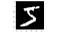Figure 21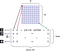Figure 22
`y`
`'5'`
`Xhat /= 255`
`pca = PCA().fit(Xhat)`
`len(pca.explained_variance_[pca.explained_variance_ <= 1e-15])`
`71`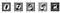Figure 23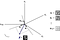Figure 24
`coordinates = pca.transform(Xhat)`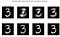Figure 25
`# Listing 23plt.plot(range(1, 785), np.cumsum(pca.explained_variance_ratio_), marker=”o”)plt.xlabel(‘Number of components’, fontsize=14)plt.ylabel(‘Explained variance ratio’, fontsize=14)plt.show()`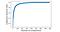Figure 26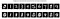Figure 27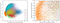Figure 28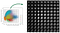Figure 29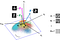Figure 30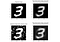Figure 31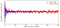Figure 32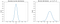Figure 33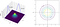Figure 34
`np.random.seed(0)mu = [2, 2]Sigma = [[6, 4],         [4, 6]]points = np.random.multivariate_normal(mu, Sigma, 150)np.round(np.cov(points.T), 2)`
`array([[5.82, 4.13],       [4.13, 6.39]])`
`points = np.random.multivariate_normal(mu, Sigma, 30000)np.round(np.cov(points.T), 2)`
`array([[6.03, 4.02],       [4.02, 5.96]])`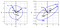Figure 35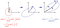Figure 36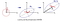Figure 37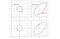Figure 38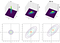Figure 39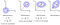Figure 40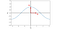Figure 41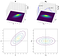Figure 42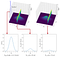Figure 43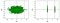Figure 44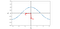Figure 45

## More from Reza Bagheri

Data Scientist and Researcher. LinkedIn: https://www.linkedin.com/in/reza-bagheri-71882a76/